# Calculate total costs based on Lookup Extra Field

Hey all,
I want to do a pretty basic thing but I don't know exactly how I can achieve it.
Basically I have a Lookup Field where I want to be able to select multiple values.There is an extra field with the price of the selected articles.
I want to show the user (readonly) the total costs of his order and save the total costs on save.

For example in the following screenshots the total cost should be displayed as 185€ and that value should also be saved in the list column "total costs".

Is there an easy way to do this?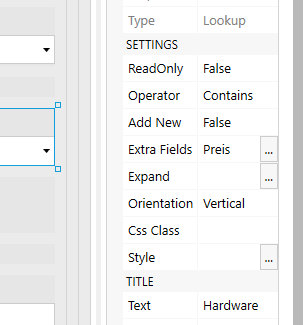Hey,
with this tutorial I now got so far, that I can select articles from my "Preisliste"-list.

Now I can't get it to work, that the price, which is there for each article in the "Preisliste"-list is also shown in the datatable.
I want to populate the "total-cost" column based on the price * quantity and save that value to my list so that I can work with the value in the SP Designer 2013 workflow.

Also another question: if I want to work with data from the datatable, for example send it via mail, it looks like this.

Is there any way to change that?

Dear @JonHebbe,
Good questions, sadly too many to answer at onceLet's start with the DataTable data. It's stored as JSON, and should be parsed first, before it can be used. For example, this can be done with MS Power Automate, with Data Operations - Parse JSON action (schema can be generated from sample value):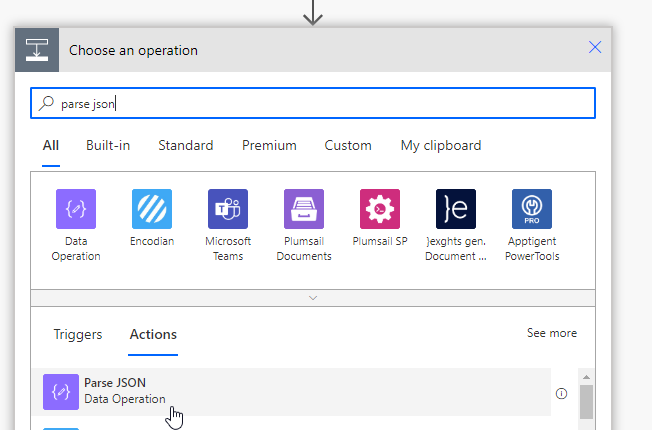It is quite a bit trickier in SharePoint Workflows, I would recommend using Power Automate flows.

Have you looked at this article, for the DataTable? - Work with Data Table using JS on Plumsail Forms for SharePoint — SharePoint forms

As for the lookup, you'll need to loop through all the values on change, and get the value of the extra field, sum them up in a separate variable, and save to another field.

Thank you very much, I got it working with the following code - I just use a normal lookup field, no DataTable right now and it works perfectly fine``````   fd.spRendered(function() {
function changeTotalCost() {
fd.field('Gesamtkosten').value = 0;
for (let i = 0; i < 30; i++) {
fd.field('Gesamtkosten').value += fd.field('Hardware').value[i].Preis;
}
}
fd.field('Hardware').\$on('change',changeTotalCost);
});

fd.spBeforeSave(function(spForm) {

fd.field('Gesamtpreis').value = fd.field('Gesamtkosten').value;

return fd._vue.\$nextTick();

});
``````

Now I got one more question where I have a problem:
In the lookup list I also have a yes/no field which says if the specific item needs approval or not. It is possible to select multiple items from that list.

Now I want to set a value in my list based on the approval value. If at least one item the user picks is marked for "needs approval" I want to flag the item as approval needed so that I can work with that value in my SP 2013 workflow.
I tried by editing my for loop but it didn't work out as expected. Can you maybe lead me to a solution?

Dear @JonHebbe,
Shouldn't be hard, if the Yes/No field is added to Extra Fields properties, you should also be able to check it's value in code:

``````fd.field('Gesamtkosten').value += fd.field('Hardware').value[i].Preis;
if(!fd.field('Hardware').value[i].Approved){
fd.field('Title').value = 'Needs approval!';
}``````

Hey, unfortunately that didn't work like expected. So I just redesigned the price list of the items so that the value "approval" is set to 0 or 1 and just calculate the total of all items. If > 0 then the manager has to approve the order.

Now my very last question (I promise)

I use
for (let i = 0; i < 30; i++)
with the static number of 30 (currently the list only has like 10 items). Here I want to put in the length of my lookup list so that I don't run into problems in the future. Is there any way I can get that number via Plumsail?

Dear @JonHebbe,
Yes, that confused me to be honest, you can just use this:

``````for (var i = 0; i < fd.field('Hardware').value.length; i++) {
fd.field('Gesamtkosten').value += fd.field('Hardware').value[i].Preis;
}``````

Thank you very much for your fast and reliable answers! It work's perfectly as expected.1 Like

I'm trying to do something similar here but am having trouble with my JS (which is why I just added it here).

I have a lookup list that includes UpgradeParts and their associated CustomerCost values, and I am trying to sum all costs from the CustomerCost values into a SP field called UpgradeCost. I don't have more than 30 items, so I left JonHebbe's value of 30 iterations.

Basically, I want to sum up the cost of any selected parts from the related list and write it to a field.

``````// Calculate Unit Cost
fd.spRendered(function() {
function calcUnitCost() {
for (let i = 0; i < 30; i++) {
}
}
});

``````

I'm getting a TypeError in my consold of "Cannot read properties of undefined" but I'm not sure how to troubleshoot that.

I believe I've aded the extra field CustomerCost (currency type SP field) correctly: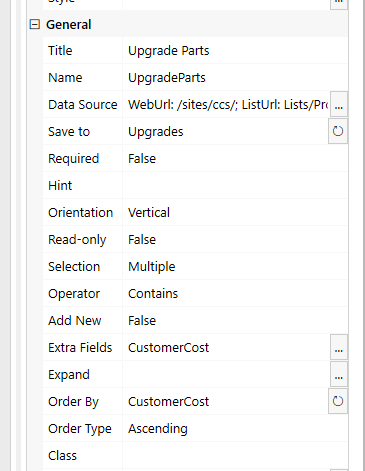Dear @GGiacomo,
Well, for one, this doesn't appear like you're using a Lookup field, but rather a Lookup control, so it should likely be:

``````fd.control('UpgradeParts')...
``````

You might also need to use ready() event - Lookup control — SharePoint forms

Thanks Nikita! The issue was the control vs. field, and I've added in the .ready function as well.

I'm now trying to add in an "if" statement to add an additional cost based on 2 different fields and I think I'm running into formatting errors.

It calculates properly when I comment out my "if" statement, so it's something in there but I can't figure out why/where it's having issues. The logic I'm trying to get: i

1. If my SharePoint choice field 'OCONUS' is equal to 'OCONUS', continue
2. Add a specific integer value to the 'UpgradeCost' field (after options have been calculated) based on the selected SharePoint choice field 'ProductCategory' (3 options)

My current JS is below:

``````// Calculate Unit Cost
fd.spRendered(function() {

// alert selected option as a text string
});

function calcUnitCost() {
for (let i = 0; i < 30; i++) {
}

// Add OCONUS cost to base
if (fd.field('OCONUS').value == 'OCONUS') {
if(fd.field('ProductCategory').value == 'Ultra-Small Desktop (UDT)') {
}
else if(fd.field('ProductCategory').value == 'High Performance Workstation (HPW)') {
}
else if(fd.field('ProductCategory').value == 'Office Desktop - SFF (ODT-SFF)') {
}
}
};

// Run calcUnitCost on UpgradeParts or OCONUS change
fd.field('OCONUS').\$on('change',calcUnitCost);
// Run calcUnitCost on form load
calcUnitCost();

});
``````

Thanks as always!

Dear @GGiacomo,
Yes, the assigned value must be on the right:

``````fd.field('UpgradeCost').value = costVar + 50;
``````

Ah ok - that cleared that error, but it's still not adding the set values (50, 120, 60) to the 'UpgradeCost' field as I would expect. Any other ideas on why they wouldn't be summing up?

Dear @GGiacomo,
What happens if you set it directly, like this?

``````fd.field('UpgradeCost').value = 50;
``````

Hey @Nikita_Kurguzov -
I am able to set the 'UpgradeCost' value directly, but not within my if() logic. Also, I switched 'UpgradeCost' to 'UnitCost' which is my SharePoint number field I'd be writing to anyways.

I modified my code a little to check 2 conditions within each If() statement instead of using nested if logic.

What I'd like to happen:

1. Add item to the 'ProductCategory' related list control and sum the cost of all items currently selected in that field (currently working correctly)
2. Review the 'OCONUS' SharePoint choice field and 'ProductCategory' choice field, and increase the number value of 'UpgradeCost' calculated in step 1 by a specific amount (currently having trouble with this)
3. Multiply the 'UnitCost' field resulting in step 2 and write it to 'TotalCost' number field (not yet written into the script)
4. Trigger this action any time 'UpgradeParts' control, 'OCONUS' field, or 'Count' field changes values and on form load

Right now, the options are summing up correctly, but there seems to be a bug in my if() logic:

``````fd.spRendered(function(){
// alert selected option as a text string
});
// calculate upgrade cost from ProductOptionsLookup list and set costVar variable for calculations
var optionSum = 0;
fd.field('UnitCost').clear();
for (let i = 0; i < 20; i++) {
}
// check OCONUS and ProductCateogry values & update cost accordingly
if (fd.field('OCONUS').value == 'OCONUS' && fd.field('ProductCategory').value == 'Ultra-Small Desktop (UDT)') {
fd.field('UnitCost').value += 1110.55;
}
else if(fd.field('OCONUS').value == 'OCONUS' && fd.field('ProductCategory').value == 'High Performance Workstation (HPW)') {
fd.field('UnitCost').value += 4088.84;
}
else if (fd.field('OCONUS').value == 'OCONUS' && fd.field('ProductCategory').value == 'Office Desktop - SFF (ODT-SFF)') {
fd.field('UnitCost').value += 1131.26;
}
else if (fd.field('OCONUS').value == 'CONUS' && fd.field('ProductCategory').value == 'Ultra-Small Desktop (UDT)') {
fd.field('UnitCost').value += 1060.55;
}
else if (fd.field('OCONUS').value == 'CONUS' && fd.field('ProductCategory').value == 'High Performance Workstation (HPW)') {
fd.field('UnitCost').value += 3938.84;
}
else if (fd.field('OCONUS').value == 'CONUS' && fd.field('ProductCategory').value == 'Office Desktop - SFF (ODT-SFF)') {
fd.field('UnitCost').value += 1071.26;
}
else {}
}

});
``````

Sorry for the lengthly repsonse and thanks as always for the help.

Dear @GGiacomo,
You need to check field name and field type. The if() conditions should work for Choice field, but will not work for other field types.

Try adding the following before the if() block and check the values in console:

``````console.log(fd.field('OCONUS').value);
console.log(fd.field('ProductCategory').value);``````

I've double checked the system names and types of both fields, and they match what I have in the code.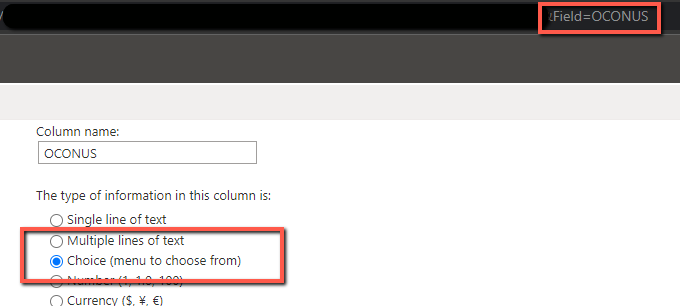Would having them be radio buttons vs. drop-downs have any impact? I wouldn't think so but figured I would ask.

I added the console.log lines but am not seeing anything in my browser console even with verbose logging - the only error (I believe) is due to the lookup code from the original post.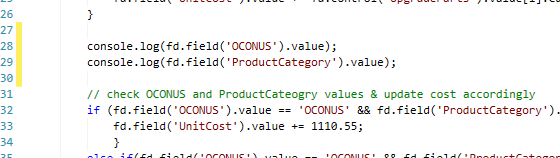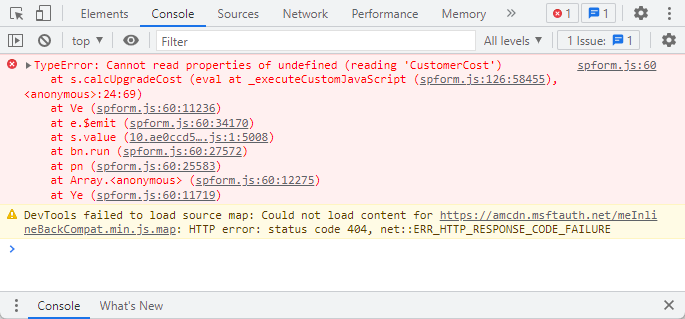Dear @GGiacomo,
The code might not execute beyond the error, so make sure to remove the parts that cause the error first, then check again - it should give you values and most likely the other parts of the code will work.

Hey @Nikita_Kurguzov - you were correct on the error killing the rest of the workflow. Since it was I added that step to the bottom of my calcUpgradeCost function (rather than trying to fix the error) and it's all calculating properly now.

I'll probably need to figure out the error sooner or later but I've got other things to work on in the meantime =)

Thanks again!